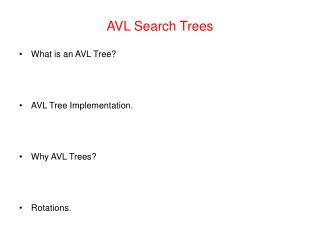DownloadDownload PresentationAVL Search Trees

# AVL Search Trees

Télécharger la présentation## AVL Search Trees

- - - - - - - - - - - - - - - - - - - - - - - - - - - E N D - - - - - - - - - - - - - - - - - - - - - - - - - - -
##### Presentation Transcript

1. AVL Search Trees • What is an AVL Tree? • AVL Tree Implementation. • Why AVL Trees? • Rotations.

2. What is an AVL Tree? • An AVL tree is a binary search tree with a height balance property: • For each node v, the heights of the subtrees of v differ by at most 1. • A subtree of an AVL tree is also an AVL tree. • For each node of an AVL tree: Balance factor = height(right subtree) - height(left subtree) • An AVL node can have a balance factor of -1, 0, or +1. -1 -1 7 7 -2 1 -1 1 3 10 3 10 0 1 13 1 0 1 4 13 1 0 Not an AVL Tree AVL Tree 2 0 2 0

3. AVL Trees Implementation public class AVLTree extends BinarySearchTree{ protected int height; public AVLTree(){ height = -1;} public int getHeight(){ return height } ; protected void adjustHeight(){ if(isEmpty()) height = -1; else height = 1 + Math.max(left.getHeight() , right.getHeight()); } protected int getBalanceFactor(){ if( isEmpty()) return 0; else return right.getHeight() - left.getHeight(); } // . . . }

4. Why AVL Trees? • Insertion or deletion in an ordinary Binary Search Tree can cause large imbalances. • In the worst case searching an imbalanced Binary Search Tree is O(n). • An AVL tree is rebalanced after each insertion or deletion. • The height-balance property ensures that the height of an AVL tree with n nodes is O(log n). • Searching, insertion, and deletion are all O(log n).

5. What is a Rotation? • A rotation is a process of switching children and parents among two or three adjacent nodes to restore balance to a tree. • An insertion or deletion may cause an imbalance in an AVL tree. • The deepest node, which is an ancestor of a deleted or an inserted node, and whose balance factor has changed to -2 or +2 requires rotation to rebalance the tree. -1 -2 50 50 Insert 35 -1 -2 45 45 78 78 0 0 0 -1 40 40 0 Deepest unbalanced node 35

6. What is a Rotation? (contd.) -2 -1 50 50 • There are two kinds of single rotation: Right Rotation. Left Rotation. • A double right-left rotation is a right rotation followed by a left rotation. • A double left-right rotation is a left rotation followed by a right rotation. rotation -2 0 45 40 78 78 0 0 -1 40 0 0 35 45 0 35

7. deepest unbalanced node a right rotation of x about y y x x y T3 T1 T2 T2 T1 T3 Single Right Rotation • Single right rotation: • The left child x of a node y becomes y's parent. • y becomes the right child of x. • The right child T2 of x, if any, becomes the left child of y. Note: The pivot of the rotation is the deepest unbalanced node

8. deepest unbalanced node a left rotation of y about x x y y x T1 T3 T2 T2 T3 T1 Single Left Rotation • Single left rotation: • The right child y of a node x becomes x's parent. • x becomes the left child of y. • The left child T2 of y, if any, becomes the right child of x. Note: The pivot of the rotation is the deepest unbalanced node

9. Single Right Rotation Implementation protected void rightRotate(){ if( isEmpty()) throw new InvalidOperationException(); BinaryTree temp = right; right = left; left = right.left; right.left = right.right; right.right = temp; Object tmpObj = key; key = right.key; right.key = tempObj; getRightAVL().adjustHeight(); adjustHeight(); }

10. Single Right Rotation Implementation (example)

11. Single Right Rotation Implementation (example) contd

12. Single Right Rotation Implementation (example) contd

13. Single Right Rotation Implementation (example) contd

14. Single Right Rotation Implementation (example) contd

15. Single Right Rotation Implementation (example) contd

16. Single Right Rotation Implementation (example) contd

17. Single Right Rotation Implementation (example) contd

18. Single Right Rotation Implementation (example) contd

19. Single Right Rotation Implementation (example) contd

20. Double Right-Left Rotation x deepest unbalanced node x y z right rotation of y about z T1 T1 y z T2 T4 T2 T3 T3 T4 y left rotation of Y about X Note: First pivot is the right child of the deepest unbalanced node; second pivot is the deepest unbalanced node x z T1 T2 T3 T4

21. x w T4 v T3 T2 T1 Double Left-Right Rotation x deepest unbalanced node v left rotation of w about v T4 w T1 T2 T3 w left rotation of W about X Note: First pivot is the left child of the deepest unbalanced node; second pivot is the deepest unbalanced node v x T1 T2 T3 T4

22. Double Rotation implementation 1 protected void rotateRightLeft() 2 { 3 if( isEmpty()) 4 throw new InvalidOperationException(); 5 getRightAVL().rotateRight(); 6 rotateLeft(); 7 } 1 protected void rotateLeftRight() 2 { 3 if( isEmpty()) 4 throw new InvalidOperationException(); 5 getLeftAVL().rotateLeft(); 6 rotateRight(); 7 }

23. x y y x T1 T3 T2 T2 T3 T1 BST ordering property after a rotation • A rotation does not affect the ordering property of a BST (Binary Search Tree). a right rotation of x about y BST ordering property requirement: BST ordering property requirement: T1 < x < y T1 < x < y x < T2 < y Similar x < T2 < y x < y < T3 x < y < T3 • Similarly for a left rotation.